# How to Calculate Combined Standard Deviation in Microsoft Excel

In this article, we will learn how to calculate combined standard deviations in Microsoft Excel. For instance, we have three distinct sets of data points. The goal is to calculate the combined standard deviation of these three datasets. By combining the datasets and calculating their combined standard deviation, we can gain insights into the overall variability or dispersion of the data as a whole.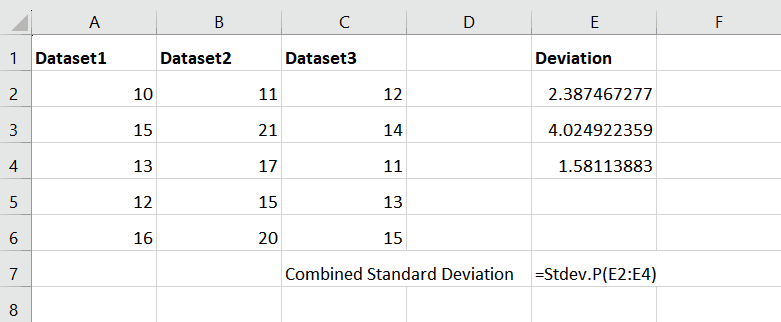The term “combined standard deviation” typically refers to the calculation of the standard deviation for a combined dataset that includes multiple individual datasets. It is useful when you want to determine the overall variability or dispersion of the combined data.

### Step 1 – Utilize STDEV Function on the First Set of Points– Choose a blank cell where you aim to apply the STDEV function.
– Now enter the Formula
=STDEV(A2:A6)
– Where the range A2:A6 contains the data points.
– The formula “=STDEV(A2:A6)” calculates the standard deviation for a single dataset

### Step 2 – Press Enter Key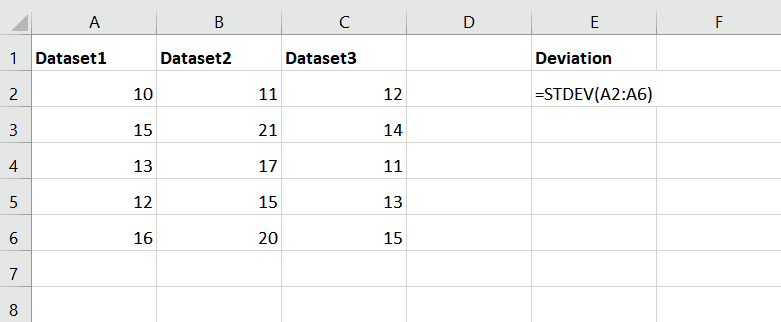– After typing Formula, press the Enter Key, and it will give you the Standard Deviation of Dataset1

### Step 3 – Utilize STDEV Function for the Second Set of Points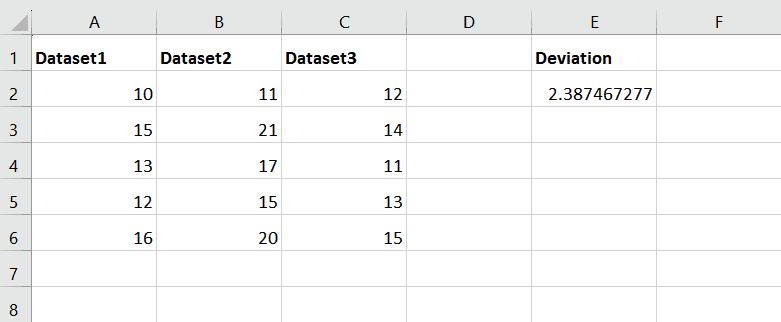– Now type the Formula “=STDEV(B2:B6)” in cell E3
– The formula “=STDEV(B2:B6)” calculates the standard deviation for a single dataset

### Step 4 – Press Enter Key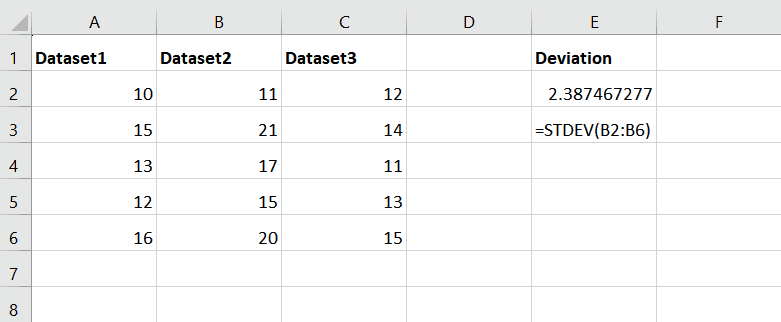– After typing Formula, press the Enter Key, and it will give you the Standard Deviation of Dataset2

### Step 5 – Utilize STDEV Function on the Third Set of Points– Now type the Formula”=STDEV(C2:C6)” in cell E4
– The formula “=STDEV(C2:C6)” calculates the standard deviation for a single dataset

### Step 6 – Press Enter Key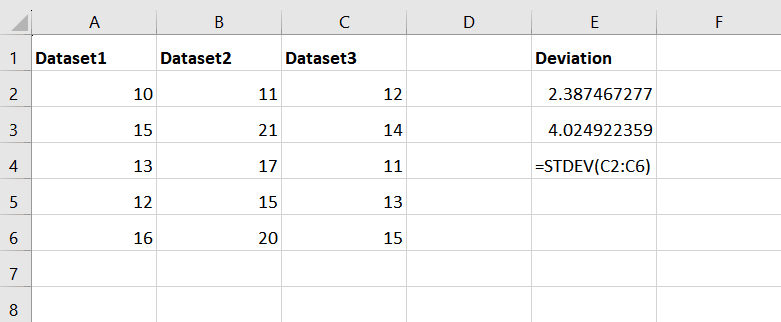– After typing Formula, press the Enter Key, and it will give you the Standard Deviation of Dataset3

### Step 7 – Now Utilize the STDEV.P Function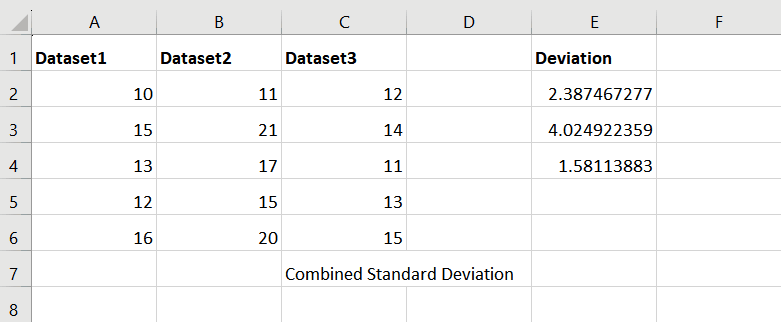– Afterward, utilize the STDEV.P function to extract the combined standard deviations.
– The structure of the function would be:
STDEV.P(E2:E4)
– Where the range E2:E4 holds the standard deviation for each distinct set.
– This function calculates the standard deviation for a population, rather than a sample. The range E2:E4 refers to the dataset or values for which you want to calculate the combined standard deviation

### Step 8 – Press Enter Key– After typing Formula, press the Enter Key, and it will give you the Combined Standard Deviation of all the datasets.